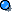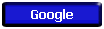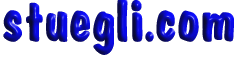#You may write just about anything you want as long as you use words and sentences rather than formulas and/or mathematical symbols. You may include definitions, concepts, rules, examples, diagrams and units.  The only restrictions will be the following: ·        No symbolic formulas or mathematical symbols (such as =,+,-,/,x) You may not write: ‘F=ma’ or ‘Force = Mass x Acceleration’ But you may write: ‘Force equals mass times acceleration’ or ‘the product of mass and acceleration is Force’ ·        You may not write exact, word for word, copies of the take-home problems, their number, or their numeric solutions (But you may include solutions in state in general terms ) Example: For the question from chapter 8 about force required to push 100kg 8m up a ramp 2m high You may not write ‘F=2m(100kg)(10)/8 = 1000/4=250 N’ But you may write: ‘Force to push an object up a ramp is equal to the weight times the height divided by the length of the ramp’Copyright © 2005 -  S. B. EglI